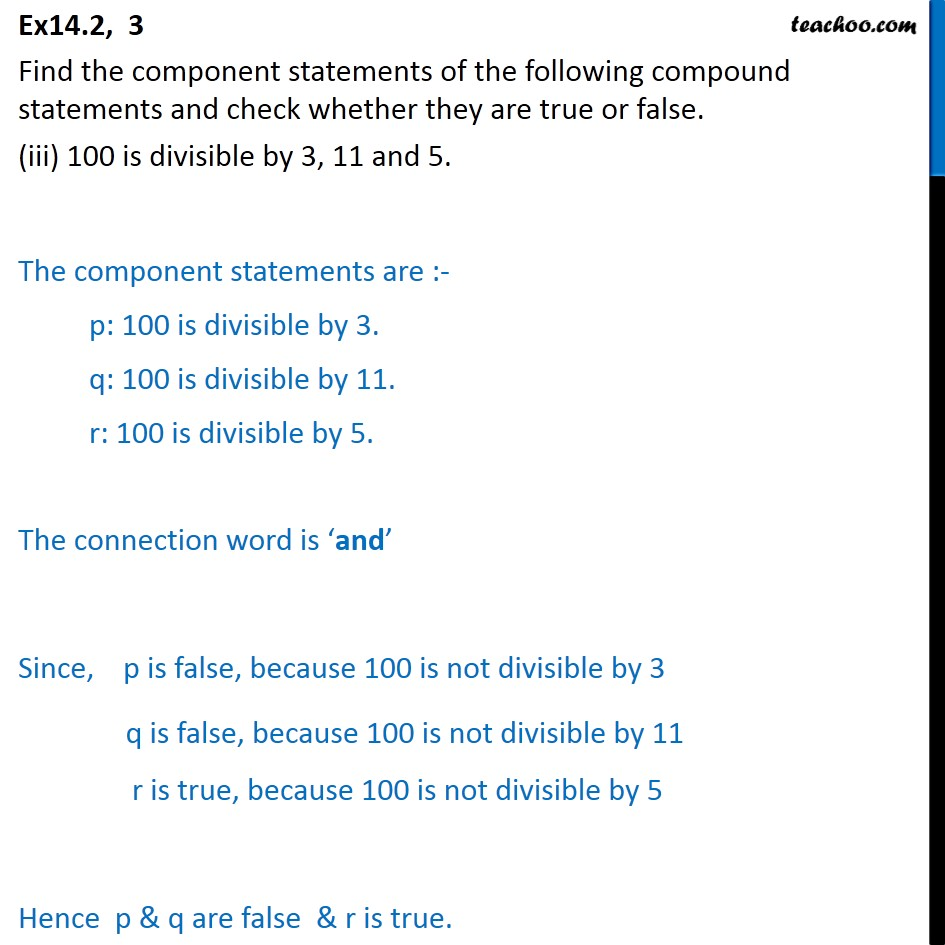Ex 14.2

Chapter 14 Class 11 Mathematical Reasoning
Serial order wiseMaths Crash Course - Live lectures + all videos + Real time Doubt solving!

### Transcript

Ex14.2, 3 Find the component statements of the following compound statements and check whether they are true or false. (iii) 100 is divisible by 3, 11 and 5. The component statements are :- p: 100 is divisible by 3. q: 100 is divisible by 11. r: 100 is divisible by 5. The connection word is ‘and’ Since, p is false, because 100 is not divisible by 3 q is false, because 100 is not divisible by 11 r is true, because 100 is not divisible by 5 Hence p & q are false & r is true.